# Point Slope Form 4 3 Slope Intercept Form

• Slides: 13Point Slope Form 4. 3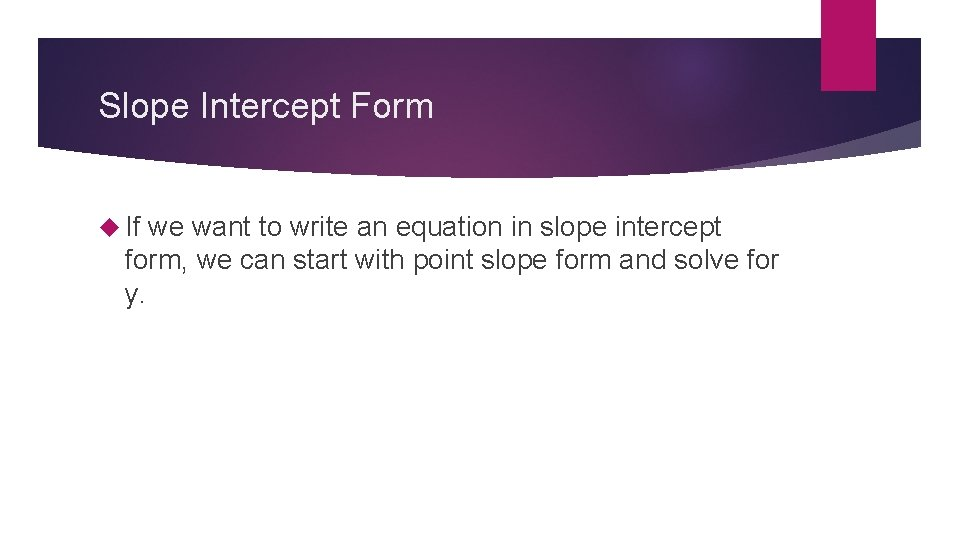Slope Intercept Form If we want to write an equation in slope intercept form, we can start with point slope form and solve for y.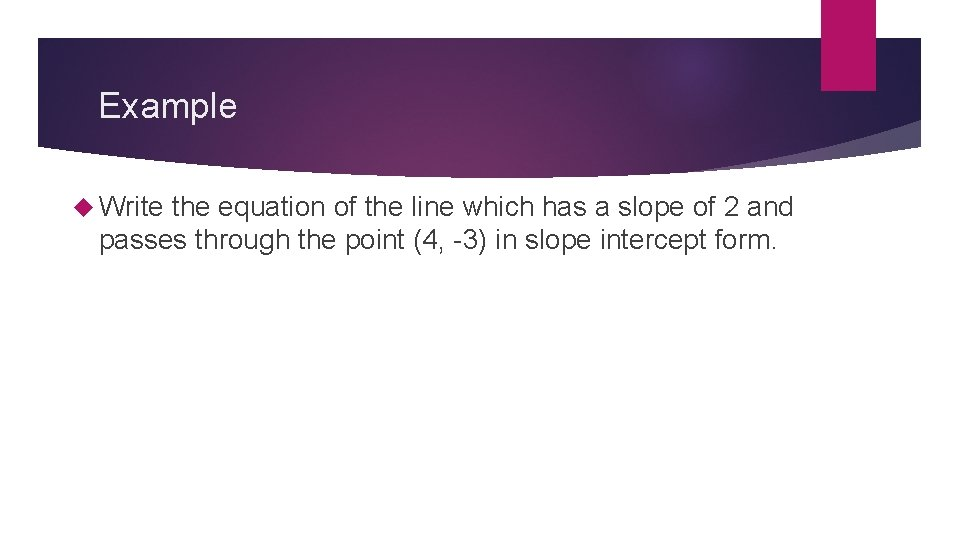Example Write the equation of the line which has a slope of 2 and passes through the point (4, -3) in slope intercept form.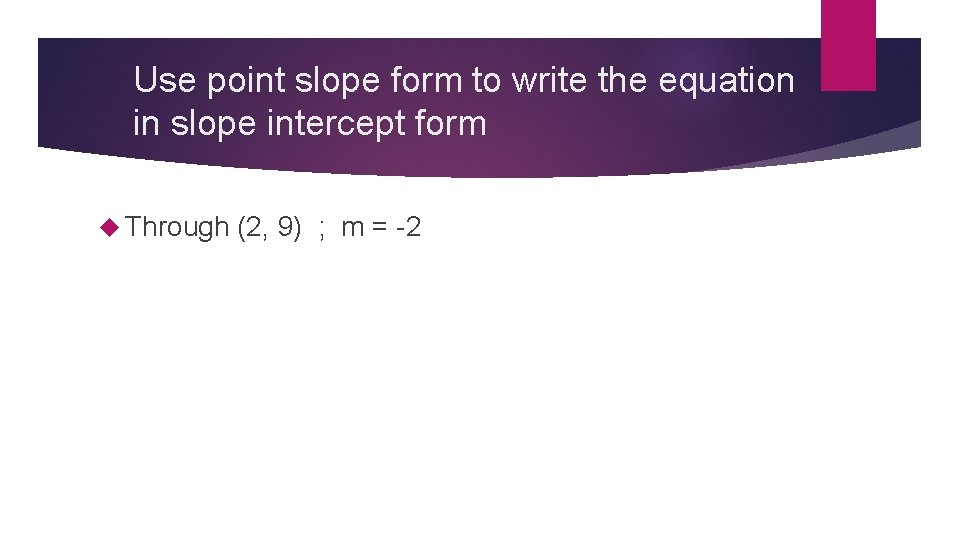Use point slope form to write the equation in slope intercept form Through (2, 9) ; m = -2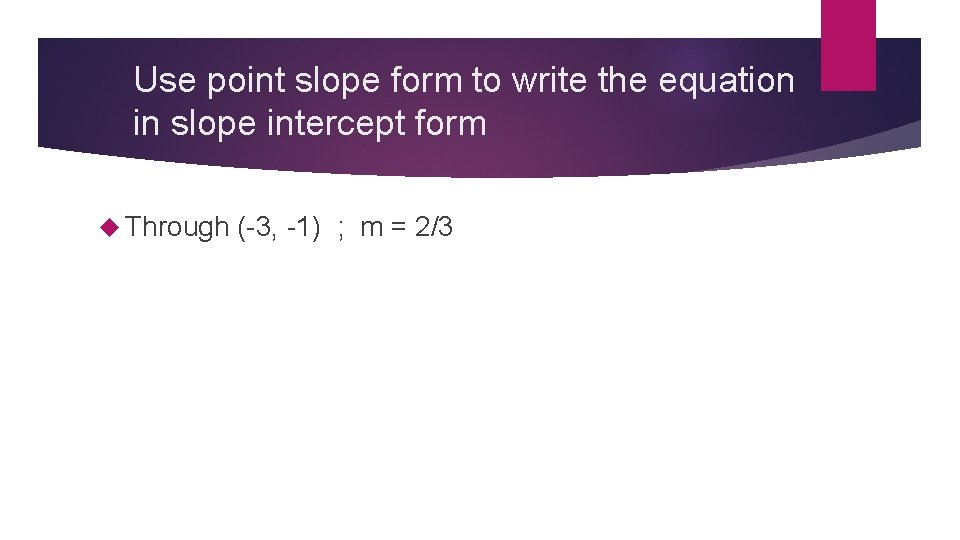Use point slope form to write the equation in slope intercept form Through (-3, -1) ; m = 2/3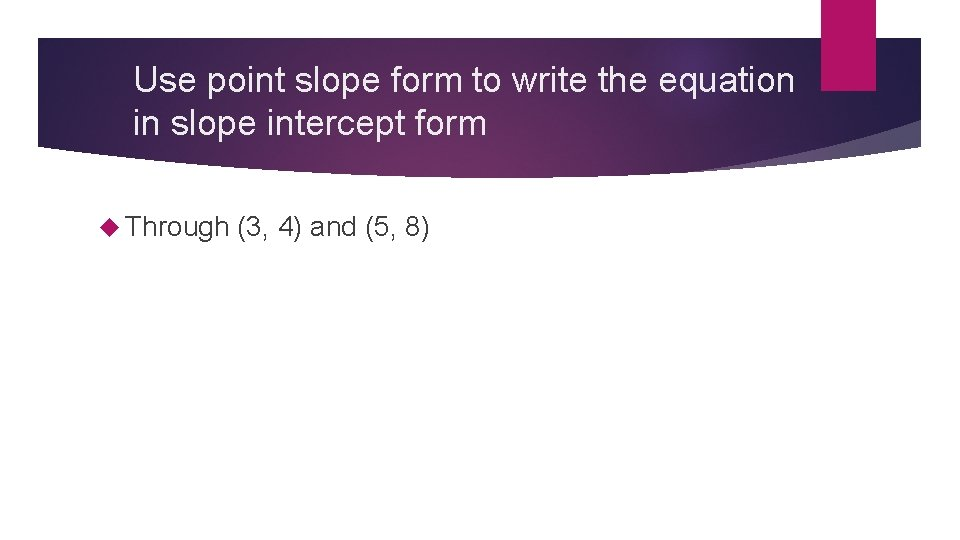Use point slope form to write the equation in slope intercept form Through (3, 4) and (5, 8)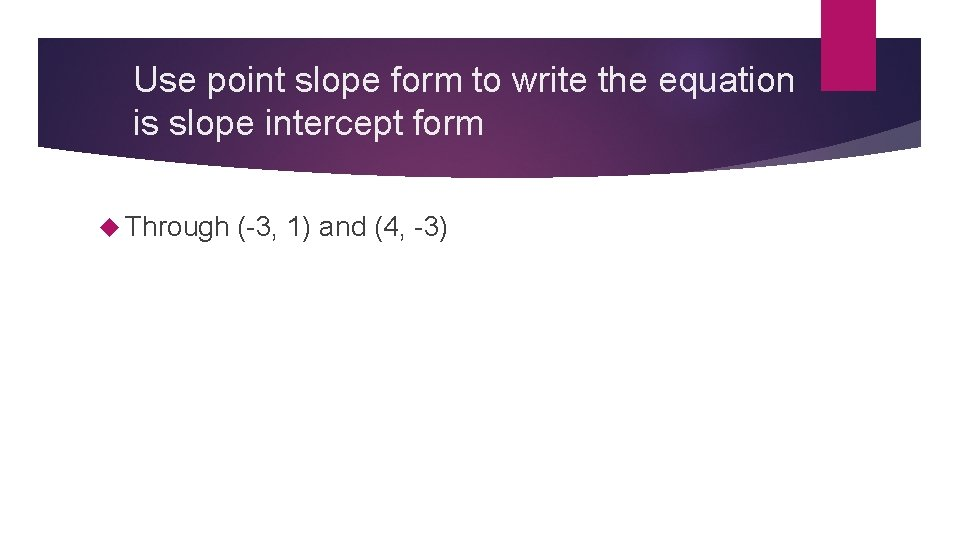Use point slope form to write the equation is slope intercept form Through (-3, 1) and (4, -3)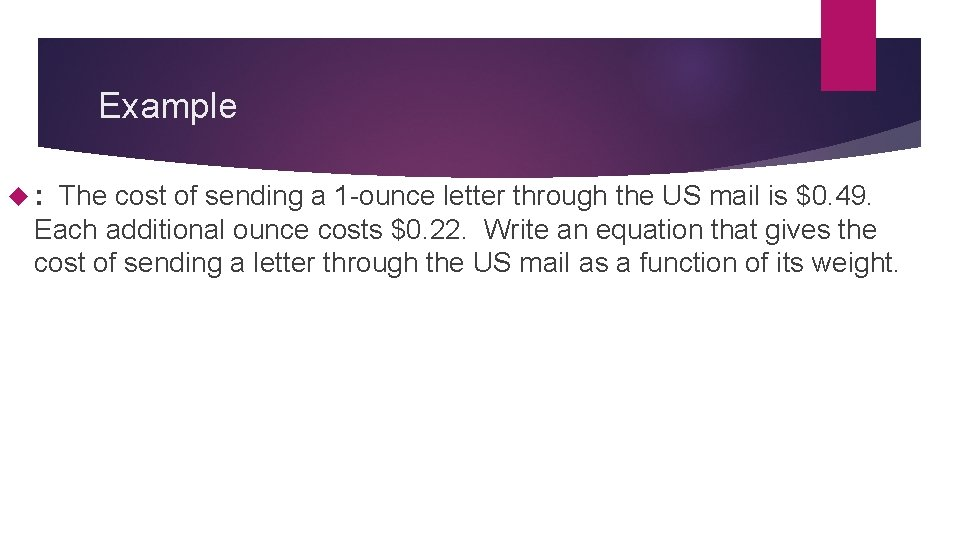Example : The cost of sending a 1 -ounce letter through the US mail is \$0. 49. Each additional ounce costs \$0. 22. Write an equation that gives the cost of sending a letter through the US mail as a function of its weight.Example Mrs. Crabb wants to order some new shirts on sale from Lands End. The cost of each shirt is \$13. 65. She will also have to pay a shipping fee. If Mrs. Crabb orders 3 shirts, the total cost will be \$48. 95. Write an equation that gives the total cost of her order as a function of the number of shirts ordered.Can the table be modeled by a linear equation? If so write the equation in point slope formCan the table be modeled by a linear equation? If so write the equation in point slope form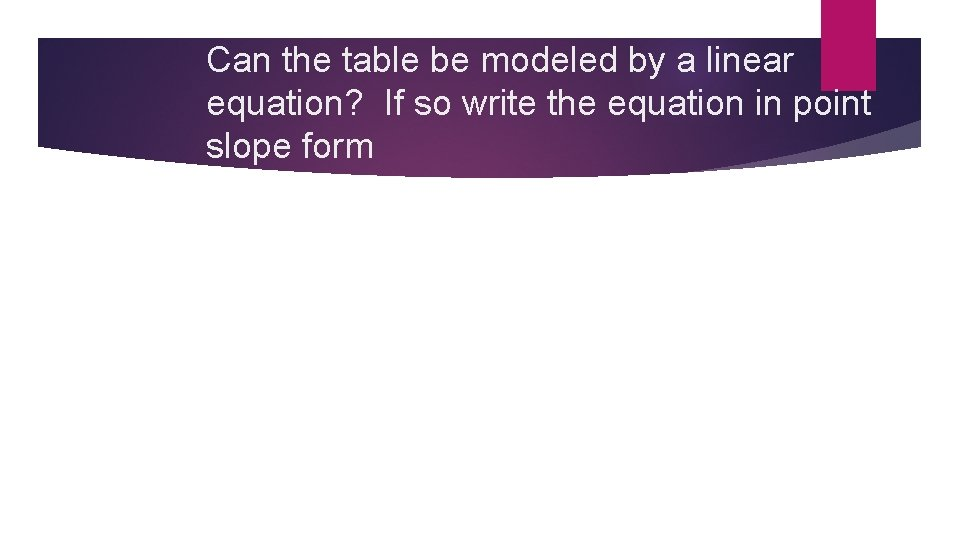Can the table be modeled by a linear equation? If so write the equation in point slope formHomework Pg 246 -247 #31 -34 all, 37, 41, 43 and the 4 additional problems from the handout.